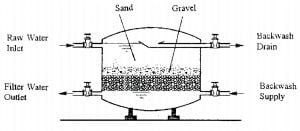Monday, October 2, 2023

# Filter Numerical Questions Slow & Rapid sand Filter Water Supply Engineering

### 1. Formula

 Surface Area Required = Q/Rate of filtration

 Geometrical Method Pn = Po(1+r/100)n

 Q = A/V### 2. Filter Numerical

1. Determine the amount of bleaching powder required annually in a water treatment plant treating 10 MLD of water if 0.3 ppm of chlorine dosage is required. Available bleaching powder contains 27% of chlorine.

Solution:

Flow Q = 10 x 106 lit/day = 10000 m3/day

Dosage= 0.3 mg/lit

Chlorine required= Flow x Dosage

= 0.3 x 10-6 kg/lit x 10 x 106 lit/day

= 3 kg/day

Bleaching powder required= 3/0.27

= 11.11 kg/day

Bleaching powder required = 4.05 tones

2. Chlorine usage in the treatment of 25 MLD of water is 9 kg/day. The residual chlorine after 10 minutes of contact is 0.2 mg/lit. Calculate the dosage in mg/lit and chlorine demand of water.

Solution:

Flow of water (Q) = 25 x 106 lit/day = 25000 m3/day

Quantity of chlorine used = 9 kg/day = 9×106 mg/day

Dosage or chlorine used= 9×106 / 25×106 = 0.36 mg/lit

Chlorine demand = Dosage – Residual chlorine

= (0.36-0.2) mg/lit

= 0.16 mg/lit

3. Design slow sand filter beds from the following data:

Population to be served= 60,000 nos

Average rate of demand= 160 lpcd

Rate of filtration= 150 liters/hr/m2

L:B = 2:1

Assume design discharge as twice the average flow and;

Assume that one unit will be kept on standby.

Solution:

The average quantity of water to be treated = 60,000 x 160 lpcd

= 96,00,000 lit/day

Design discharge (Q) = 2x 96,00,000 lit/day

= 19.2 x 106 lit/day

Rate of filtration= 150 liters/hr/m2

= 24 x 150 = 3600 liters/day/m2

Surface area required= Q/ Rate of filtration

= 19.2×106/3600

=5333.34 m2

Assuming 5 filter units;

Area for each filter bed(as) = 5333.34/5 = 1066.67 m2

As = L x B = 2B2 = 1066.67

B= √1066.66/2

B= 23.09 m

L= 46.02 m

Length (L) = 46.02m

Providinf freeboard = 0.5 m, water depth=1 m, depth filter media = 1m, depth of base material= 0.6, depth of under drain pipe= 0.2 m, therefore overall depth of filter= 3.30

Providing one filter unit standby, required filter units= 6 with a dimension of 46.02 m x 23.10 m x 3.30 m.

 Read More: Hardness and Alkalinity Numerical

4. Average water consumption rate is 150 lpcd in an urban area. Design a slow sand filter for a community having a population of 10000 at the base year 2068.

Solution:

Assume annual population growth rate 1.7% and design period= 15 years

Using a geometrical method for forecasting population of the design year 2083;

Geometrical Method Pn = Po(1+r/100)n

The average quantity of water to be treated,

= 12877 nos x 150 lpcd

= 1931550 lit/day

Let design discharge is equal to the average quantity of water (Q)

= 1931550 lit/day

Assuming rate of filtration = 150 liters/hr/m2

= 24 x 150 = 3600 liters/hr/m2

Surface area required= Q/Rate of filtration

= 1931550/3600

=536.54 m2

Assuming 3 filter units;

Area for each filter bed (as) = 536.54/3

= 178.84 m2

As= Lx B = 2B2 = 178.84 m2

B= √178.84/2

B= 9.5 m

L= 19 m

Breadth (B)= 9.5 m, Length (L) = 19m

Providing free board=0.5 m, water depth= 1m, depth filter media= 1m, depth of base material=0.6m, depth of under drain pipe= 0.2m. Therefore overall depth of filter= 3.30m

Providing one filter unit standby, required filter units= 4 with a dimension of 19 m x 9.5 m x 3.30 m.

5. Calculate the dimension of a set of rapid sand filters for treating water required for a population of 0.1 million with an average rate of demand of 200 lpcd.

Solution:

Average quantity of water to be treated= 0.1 x 106 x 200 lpcd

= 20 x106 lit/day

Assuming that 3 % of filtered water is required for filter backwashing and time required for backwashing= 30 minutes

Discharge (Q) = 20000000 lit/day

= 20×106 lit/day

= 833333.34 lit/hr

Design discharge (Q) = 833333.34/(1-0.03)x(24-0.5)

= 36557.72 lit/hr

Assume rate of filtration = 4000 liters/hr/m2

= 24 x 4000

= 96000 liters/day/m2

Surface area required = Q/ Rate of filtration

= 877385.39/96000

=9.13 m2

Minnimum Surface area (As) = 10 m2

Assuming,

L:B=1.3:1

As= LxB

B= 2.8 m

L= 3.65 m

Breadth (B) = 2.8 m, Length (L) = 3.65 m

Provide, freeboard=0.5 m, water depth= 1.6 m, Filter media = 0.6 , Base material = 0.6m, Under drain pipe depth= 0.2m. Therefore, overall depth = 3.5 m

Providing one filter unit standby, required filter units= 2 with the dimension of 3.65m x 2.8m x 3.5m.He is a founder and lead author of Dream Civil International and his civil engineering research articles has been taken as source by world's top news and educational sites like USA Today, Time, The richest, Wikipedia, etc.
Latest Articles

Related Articles